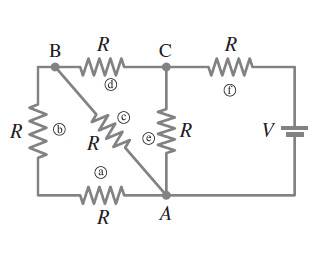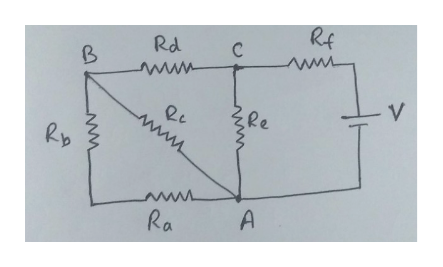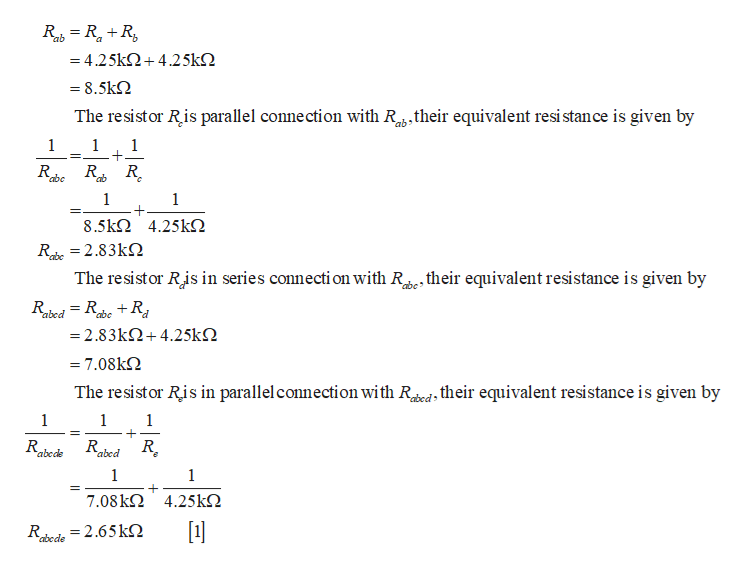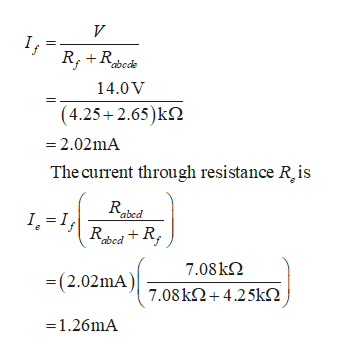RCВwwRwwR(awwR Aww

Question

The current through each resistor in  if each resistance R = 4.25 kΩ and V = 14.0 V . What is the through resistor a,b,c,d,e,f, and g?help_outlineImage TranscriptioncloseR C В ww R ww R (a ww R A ww fullscreen
Step 1

Given that the value of all the resistors is equal and has the value R=4.25kΩ.

The circuit is shown below.help_outlineImage TranscriptioncloseRf Rd www V Rp www Ra A fullscreen
Step 2

The resistor Ra and Rb are connected in series , the equivalent Resistance is given byhelp_outlineImage Transcriptioncloseab =4.25k 4.25k 8.5k2 The resistor Ris parallel connection with Ra,their equivalent resi stance is given by 1 1 1 _ R R abe ab 1 1 = 8.5k 4.25k Rte 2.83k abe The resistor Ris in series connection with R^e, their equivalent resistance is given by abe + abe abed -2.83k4.25k = 7.08k2 The resistor Ris in parallel connection with Red their equivalent resistance is given by 1 1 1 = abede abed 1 1 7.08k 4.25k abede =2.65KO fullscreen
Step 3

The equivalent resistor Rabcde  is in series connection with the resistor Rf , and current through both these...help_outlineImage TranscriptioncloseV If abede 14.0 V (4.25+2.65)k2 -2.02mA The current through resistance R is abed 1.=RedR; 7.08k = (2.02mA)o8k2+4.25k2 1.26mA fullscreen

Want to see the full answer?

See Solution

Want to see this answer and more?

Our solutions are written by experts, many with advanced degrees, and available 24/7

See Solution
Tagged in

Physics